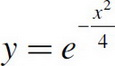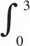# AP Calculus AB Practice Test 14

### Test Information8 questions24 minutes

Calculator Allowed

1. If f(x) is the function given by f(x) = e3x + 1, at what value of x is the slope of the tangent line to f(x) equal to 2 ?

2. The graph of the function y = x3 + 12x2 + 15x + 3 has a relative maximum at x =

3. The side of a square is increasing at a constant rate of 0.4 cm/sec. In terms of the perimeter, P, what is the rate of change of the area of the square, in cm2/sec?

4. Let f be the function given by f(x) = 3x. For what value of x is the slope of the line tangent to the curve at (x, f(x)) equal to 1 ?

5. Given f and g are differentiable functions and

f(a) = -4, g(a) = c, g(c) = 10, f(c) = 15

f′(a) = 8, g′(a) = b, g′(c) = 5, f′(c) = 6

If h(x) = f(g(x)), find h′(a)

6. What is the area of the region in the first quadrant enclosed by the graph ofand the line y = 0.5 ?

7. What is the trapezoidal approximation ofexdx using n = 4 subintervals?

8. The second derivative of a function f is given by f′′(x) = x sin x - 2. How many points of inflection does f have on the interval (-10, 10) ?